Electromagnetism Flashcards Preview

Linlithgow Academy Advanced Higher Physics > Electromagnetism > Flashcards

Flashcards in Electromagnetism Deck (29):
1

2

What would the electric field pattern be around a single point negative charge?3

What would the electric field pattern be around a single point positive charge?4

5

6

7

What would the the electric field pattern between two oppositely charged parallel plates look like? (ignore end effects).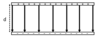8

Three similar looking relationships are shown below for E, F and V.Define what E, F and V are in terms of the charges (Q) in each relationship.9

Sketch a current against time graph for a charging capacitor in a d.c. "RC" circuit.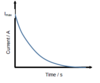10

Sketch a current against time graph for a discharging capacitor in a d.c. "RC" circuit.

(note the 'negative' current indicating a change in dirction of current flow)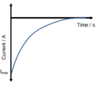11

Sketch a voltage against time graph for a discharging capacitor in a d.c. "RC" circuit.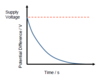12

Sketch a voltage against time graph for a charging capacitor in a d.c. "RC" circuit.13

14

How could you determine the time constant from a voltage-time graph such as this?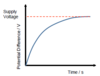15

16

17

Using the circuit shown, describe how you could determine graphically the capacitive reactance of the capacitor.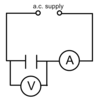Vary the a.c. power supply voltage taking readings of V and I. Plot V (y-axis) against I (x-axis) The gradient of the straight line gives Xc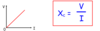18

In the circuit shown, the frequency of the a.c. current is increased steadily. Describe what happens to the value of the a.c. current in the circuit.19

In the circuit shown, the frequency of the a.c. current is increased steadily. Describe what happens to the value of the a.c. current in the circuit.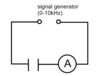The value of the a.c. current increases in direct proportion to the frequency of the supply.20

It can be shown that the value of the a.c. current in a inductive circuit is inversely proportional to the frequency of the supply.What does this tell us about the inductive reactance of the inductor as freqnecy increases?

As the frequency of the supply is increased, the current decreases. This must mean XL is increasing.This means that XL is directly proportional to the frequency of the supply.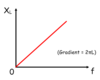21

It can be shown that the value of the a.c. current in a capactive circuit is direclty proportional to the frequency of the supply.What dos this tell us about the capacitive reactance of the capacitor as freqnecy increases?As the frequency of the supply is increased, the current increases. This must mean Xc is decreasing.This means that Xc is inversely proportional to the frequency of the supply.22

23

24

25

26

How can you determine the rate of change of current from a current-time graph such as this?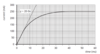27

In terms of fields, describe the nature of electromagnetic radiation

All EM waves are oscillating electric and magnetic fields at right angles to, and in phase with, each other.28

29